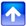# Logic Gates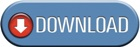Download the pdf version of these notes.

Introduction
Logic gates process signals which represent true or false. Normally, the positive supply voltage +5V represent true and 0V represents false. Other terms which are used for the true and false states are shown in the table. It is best to be familiar with them all.

 Logic States True False 1 0 High Low On Off +5V 0V

Gates are identified by their function: AND, OR, NOT, NAND, NOR, EX-OR and EX-NOR. Capital letters are normally used to make it clear that the term refers to a logic gate.

The above said logic gates can be classified into following categories:

 1. Basic Logic Gates a. AND Gate b. OR Gate c. NOT Gate 2. Universal Gates a. NAND Gate b. NOR Gate 3. Combinational Gates a. X-OR Gate b. X-NOR Gate

The basic operations are described below with the aid of truth tables.

Basic Logic Gates
AND Gate
The AND gate is an electronic circuit that gives a high output (1) only if all its inputs are high.  A dot (.) is used to show the AND operation i.e. A.B.  Bear in mind that this dot is sometimes omitted i.e. AB.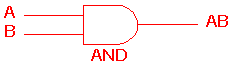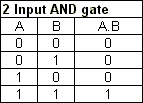OR Gate
The OR gate is an electronic circuit that gives a high output (1) if one or more of its inputs are high.  A plus (+) is used to show the OR operation.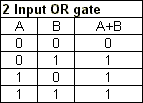NOT gate
The NOT gate is an electronic circuit that produces an inverted version of the input at its output.  It is also known as an inverter.  If the input variable is A, the inverted output is known as NOT A.  This is also shown as A', or A with a bar over the top, as shown at the outputs.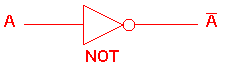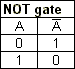Universal Gates
NAND Gate
This is a NOT-AND gate which is equal to an AND gate followed by a NOT gate.  The outputs of all NAND gates are high if any of the inputs are low. The symbol is an AND gate with a small circle on the output. The small circle represents inversion.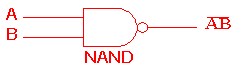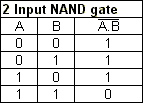NOR Gate
This is a NOT-OR gate which is equal to an OR gate followed by a NOT gate.  The outputs of all NOR gates are low if any of the inputs are high. The symbol is an OR gate with a small circle on the output. The small circle represents inversion.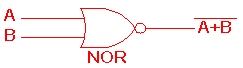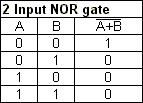Combinational Gates
X-OR Gate
The 'Exclusive-OR' gate is a circuit which will give a high output if either, but not both, of its two inputs are high.  An encircled plus sign (⊕) is used to show the X-OR operation.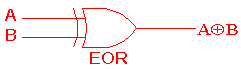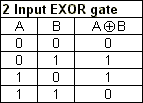X-NOR Gate
The 'Exclusive-NOR' gate circuit does the opposite to the X-OR gate. It will give a low output if either, but not both, of its two inputs are high. The symbol is an X-OR gate with a small circle on the output. The small circle represents inversion.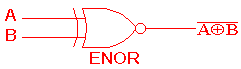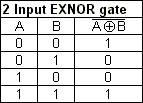Table 1: Logic Gate Symbols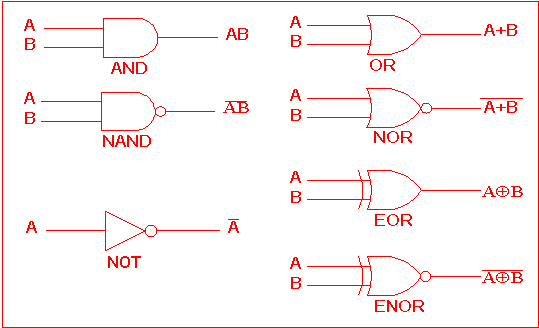Table 2 is a summary truth table of the input/output combinations for the NOT gate together with all possible input/output combinations for the other gate functions.

Table 2: Logic gates representation using the Truth table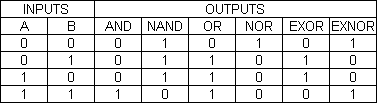W3Professors

VacationBazaar

Relevant-Education

### Our Other Websites